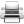# Computational & Multiscale Mechanics of Materials

## Fracture Mechanics Online Class

Other online classes : Aircraft structures

# SIF Computation

This chapter introduces the existing different methods available to compute the Stress Intensity factor (SIF), which are

• The analytical methods based on Linear Fracture Elastic Mechanics: by establishing the full field solution, by applying the superposition of existing solutions or by considering the energetic method related to Griffith work;
• The numerical approaches: based on collocation method or on FEM by using the field approximation, the energetic method of the J-integral;
• The experimental approaches: based on normalized experiments or by using the strain Gauge Method;
• The use of tabulated solutions available in handbooks.

# SIF Computation > Analytical methods: Reminder of Linear Elasticity

Under the assumptions of 2D linear elasticity, the problem can be stated in terms of the Airy function and is thus governed by the bi-harmonic equation:

\begin{equation} \nabla^2 \nabla^2 \Phi = 0. \label{eq:biharmonic}\end{equation}

One solution of this equation has the form:

\begin{equation} \Phi = \frac{\bar{\zeta}\Omega+\zeta\bar{\Omega} + \omega + \bar{\omega}}{2} ,\label{eq:PhiCrack}\end{equation}

where the functions $\omega(\zeta)$ and $\Omega(\zeta)$ have to be determined so that the boundary conditions are satisfied. The solution fields can be expressed in terms of these functions as:

• The stress field reads: \begin{equation} \left\{ \begin{array}{r c l} \mathbf{\sigma}_{xx} &= &\Omega'+\bar{\Omega}' - \frac{\bar{\zeta}\Omega''+\omega''+ \zeta\bar{\Omega}''+ \bar{\omega}''}{2},\\ \mathbf{\sigma}_{yy} &=&\Omega'+\bar{\Omega}' + \frac{\bar{\zeta}\Omega''+\omega''+ \zeta\bar{\Omega}''+ \bar{\omega}''}{2},\\ \mathbf{\sigma}_{xy} &=& i \frac{\zeta\bar{\Omega}''+\bar{\omega}''-\bar{\zeta}\Omega''-\omega''}{2}. \end{array} \right. \label{eq:stressAiry}\end{equation}
• The displacement field reads: \begin{equation} \mathbf{u} = -\frac{1+\nu}{E}\left(\zeta\bar{\Omega}'+\bar{\omega}' + \kappa \left(\zeta\right)\right),\label{eq:uAiry} \end{equation} with \begin{equation} \left\{ \begin{array}{r c l l} \kappa &=&\frac{3-\nu}{1+\nu}&\text{ in Plane} \quad \sigma,\\ \kappa &=&3-4\nu&\text{ in Plane} \quad\epsilon. \end{array} \right. \end{equation}Last update: September 02, 2015